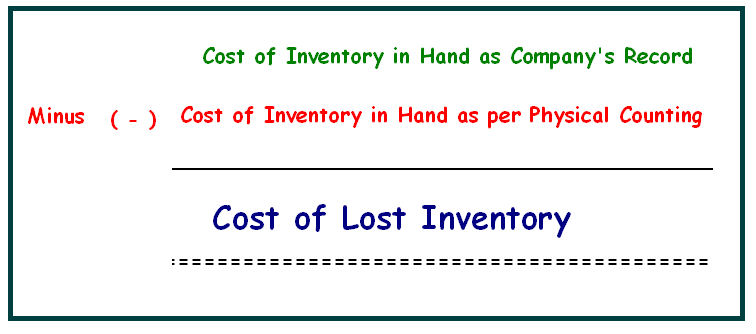If we want to improve our working capital through proper management of inventory from its buying to sale, we have to decrease the cost of lost inventory. There are lots of reasons of lost inventory like stolen by employees or misplace. So, correct calculation will help to take step to prevent all these reasons.

So, for accountants, to calculate the cost of lost inventory is must. Following is the simple formula for all who even who  know whole basic accounting.

For example : A company has inventory of \$ 50,000 on 31st March as per our accounting record which shows our accounting software. But when we count our inventory through physical counting, we found the inventory of \$ 40,000. It means, we have the inventory lost of \$ 10,000. Now, we have the issue of lost inventory. This issue may be due to missing, misplace or stealing. So, we can make the protocol to finding the reasons of lost inventory.

Above is simple formula but there is also alternative for calculating the lost inventory.

Actual Cost of Goods Sold  - (Cost of Opening  Inventory as per physical counting + Cost Purchase as per physical counting) = Actual Closing Inventory as per physical counting

Now, Find the closing stock as per record. Difference in the actual and recorded closing stock is the inventory lost.

* Important = Actual cost of goods sold can be calculated

actual sale - actual gross profit

Related :.

## \$type=three\$a=hide\$cm=hide\$s=hide\$show=/2019/06/10-steps-to-become-better-investor.html\$l=hide

Name

false
ltr
item
Accounting Education: How to Calculate Cost of Lost Inventory
How to Calculate Cost of Lost Inventory
After understanding the importance of calculation of lost inventory, we are explain its simple steps.
http://3.bp.blogspot.com/-9WLCIJ6XcF4/Uy-N8wqJFII/AAAAAAAANOE/kNfMimglovc/s1600/formula+of+lost+inventory.PNG
http://3.bp.blogspot.com/-9WLCIJ6XcF4/Uy-N8wqJFII/AAAAAAAANOE/kNfMimglovc/s72-c/formula+of+lost+inventory.PNG
Accounting Education
https://www.svtuition.org/2014/03/how-to-calculate-cost-of-lost-inventory.html
https://www.svtuition.org/
http://www.svtuition.org/
http://www.svtuition.org/2014/03/how-to-calculate-cost-of-lost-inventory.html
true
2410664366776677676
UTF-8